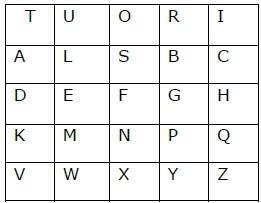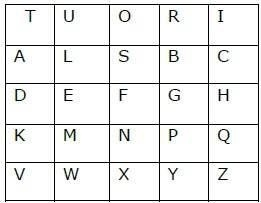C++ Program to Decode a Message Encoded Using Playfair Cipher

C++Server Side ProgrammingProgramming

In this scheme, pairs of letters are encrypted, instead of single letters as in the case of simple substitution cipher.

In playfair cipher, initially a key table is created. The key table is a 5×5 grid of alphabets that acts as the key for encrypting the plaintext. Each of the 25 alphabets must be unique and one letter of the alphabet (usually J) is omitted from the table as we need only 25 alphabets instead of 26. If the plaintext contains J, then it is replaced by I.

The sender and the receiver deicide on a particular key, say ‘tutorials’. In a key table, the first characters (going left to right) in the table is the phrase, excluding the duplicate letters. The rest of the table will be filled with the remaining letters of the alphabet, in natural order. The key table works out to be −Process of Playfair Cipher

First, a plaintext message is split into pairs of two letters (digraphs). If there is an odd number of letters, a Z is added to the last letter. Let us consider that, we want to encrypt the message “hide money”. It will be written as −

HI DE MO NE YZ

The rules of encryption are −

• If both the letters are in the same column, take the letter below each one (going back to the top if at the bottom)‘H’ and ‘I’ are in same column, hence take letter below them to replace. HI → QC• If both letters are in the same row, take the letter to the right of each one (going back to the left if at the farthest right)‘D’ and ‘E’ are in same row, hence take letter to the right of them to replace. DE → EF• If neither of the preceding two rules are true, form a rectangle with the two letters and take the letters on the horizontal opposite corner of the rectangle.Using these rules, the result of the encryption of ‘hide money’ with the key of ‘tutorials’ would be −

QC EF NU MF ZV

Decrypting the Playfair cipher is as simple as doing the same process in reverse. Receiver has the same key and can create the same key table, and then decrypt any messages made using that key.

Here is a C++ program is given to encode a message using Playfair Cipher.

Algorithms

Begin
Function void play( int dir )
For it = msg.begin() to it != msg.end()
If ( getPos( *it++, j, k ) )
If ( getPos( *it, p, q) )
If ( j == p )
nmsg+= getChar( j, k + dir )
nmsg += getChar( p, q + dir )
else if( k == q )
nmsg += getChar( j + dir, k )
nmsg += getChar( p + dir, q )
else
nmsg += getChar( p, k )
nmsg += getChar( j, q )
done
done
done
msg = nmsg
done
End

Example

#include <iostream>
#include <string>
using namespace std;
class playfair {
public:
string msg; char n;
void play( string k, string t, bool m, bool e ) {
createEncoder( k, m );
getText( t, m, e );
if( e )
play( 1 );
else
play( -1 );
print();
}
private:
void play( int dir ) {
int j,k,p,q;
string nmsg;
for( string::const_iterator it = msg.begin(); it != msg.end(); it++ ) {
if( getPos( *it++, j, k ) )
if( getPos( *it, p, q) ) {
//for same row
if( j == p ) {
nmsg+= getChar( j, k + dir );
nmsg += getChar( p, q + dir );
}
//for same column
else if( k == q ) {
nmsg += getChar( j + dir, k );
nmsg += getChar( p + dir, q );
} else {
nmsg += getChar( p, k );
nmsg += getChar( j, q );
}
}
}
msg = nmsg;
}
void print() //print the solution {
cout << "\n\n Solution:" << endl;
string::iterator it = msg.begin(); int count = 0;
while( it != msg.end() ) {
cout << *it;
it++;
cout << *it << " ";
it++;
if( ++count >= 26 )
cout << endl;
count = 0;
}
cout << endl << endl;
}
char getChar( int a, int b ) { //get the characters
return n[ (b + 5) % 5 ][ (a + 5) % 5 ];
}
bool getPos( char l, int &c, int &d ) { //get the position
for( int y = 0; y < 5; y++ )
for( int x = 0; x < 5; x++ )
if( n[y][x] == l ) {
c = x;
d= y;
return true;
}
return false;
}
void getText( string t, bool m, bool e ) { //get the original message
for( string::iterator it = t.begin(); it != t.end(); it++ ) {
//to choose J = I or no Q in the alphabet.
*it = toupper( *it );
if( *it < 65 || *it > 90 )
continue;
if( *it == 'J' && m )
*it = 'I';
else if( *it == 'Q' && !m )
continue;
msg += *it;
} if( e ) {
string nmsg = ""; size_t len = msg.length();
for( size_t x = 0; x < len; x += 2 ) {
nmsg += msg[x];
if( x + 1 < len ) {
if( msg[x] == msg[x + 1] ) nmsg += 'X';
nmsg += msg[x + 1];
}
}
msg = nmsg;
}
if( msg.length() & 1 )
msg += 'X';
}
void createEncoder( string key, bool m ) { //creation of the key table
if( key.length() < 1 )
key= "KEYWORD";
key += "ABCDEFGHIJKLMNOPQRSTUVWXYZ";
string s= "";
for( string::iterator it = key.begin(); it != key.end(); it++ ) {
*it = toupper( *it );
if( *it < 65 || *it > 90 )
continue;
if( ( *it == 'J' && m ) || ( *it == 'Q' && !m ) )
continue;
if( s.find( *it ) == -1 )
s += *it;
}
copy( s.begin(), s.end(), &n );
}
};
int main( int argc, char* argv[] ) {
string k, i, msg;
bool m, c;
cout << "Encrpty or Decypt? ";
getline( cin, i );
c = ( i == 'e' || i == 'E' );
cout << "Enter a key: ";
getline( cin, k);
cout << "I <-> J (Y/N): ";
getline( cin, i );
m = ( i == 'y' || i == 'Y' );
cout << "Enter the message: ";
getline( cin, msg );
playfair pf;
pf.play( k, msg,m, c );
return system( "pause" );
}

Output

Encrpty or Decypt? d
Enter a key: players
I <-> J (Y/N): y
Enter the message: OK GC GC MZ MQ CF YA RL QH OM

Solution:
TH IS IS TU TO RI AL SP OI NT